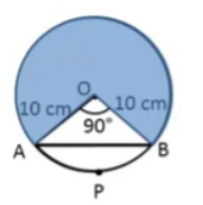A chord of a circle of radius 10cm subtends a right angle at the centre. ____ is the area of the major sector. Chapter/Topic:  circles

# A chord of a circle of radius 10cm subtends a right angle at the centre. ____ is the area of the major sector. Chapter/Topic:  circles

Fill Out the Form for Expert Academic Guidance!l

+91

Live ClassesBooksTest SeriesSelf Learning

Verify OTP Code (required)

### Solution:

In the mentioned circle,O is the centre and AO =BO = Radius = 10 cm
AB is a chord which subtents 90o at centre O, i.e., AOB=${{90}}^{{0}}$
The angle at the sector is 90 degrees so the area of the sector is equal to the quadrant of the circle.
Area of Major sector = Area of circle - Area of Sector AOB
Area of sector = Area of circle/4
= (π×10×10)−
=314−78.5
=235.5 ${{\mathit{cm}}}^{{2}}$
Hence, 235.5 ${{\mathit{cm}}}^{{2}}$ is the area of the major sector.

## Related content

 Area of Square Area of Isosceles Triangle Pythagoras Theorem Triangle Formula Perimeter of Triangle Formula Area Formulae Volume of Cone Formula Matrices and Determinants_mathematics Critical Points Solved Examples Type of relations_mathematics+91

Live ClassesBooksTest SeriesSelf Learning

Verify OTP Code (required)